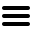vocabulary
1000+ books

# mean used in The War of the Worlds

only 1 use
(click/touch triangles for details)
Definition
the average number
For example, the mean of 1 & 3 is 2.
More generally, the mean of n numbers is computed by dividing the sum of the numbers by n; for example, the mean of 1, 2, and 6
= (1+2+6) / 3
= 9 / 3
= 3
• The planet Mars, I scarcely need remind the reader, revolves about the sun at a mean distance of 140,000,000 miles, and the light and heat it receives from the sun is barely half of that received by this world.
1.1 — Book 1 Chapter 1 — The Coming of the Martians (13% in)
mean = average
There are no more uses of "mean" in The War of the Worlds.

Typical Usage  (best examples)